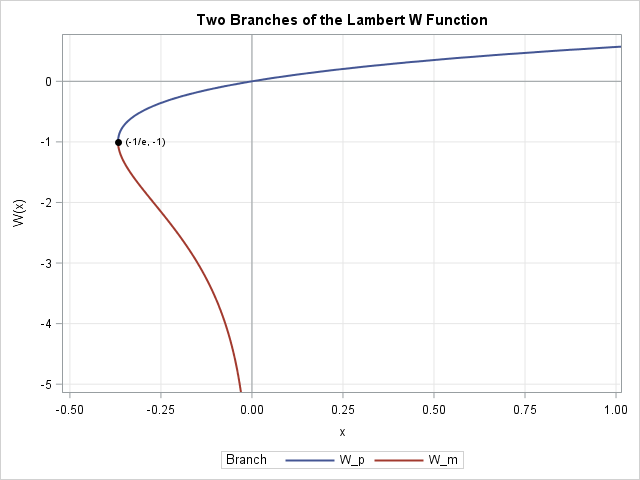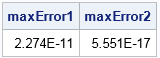This article describes how you can evaluate the Lambert W function in SAS/IML software. The Lambert W function is defined implicitly: given a real value x, the function's value w = W(x) is the value of w that satisfies the equation w exp(w) = x. Thus W is the inverse of the function g(w) = w exp(w).

Because the function g has a minimum value at w=-1, there are two branches to the Lambert W function. Both branches are shown in the adjacent graph. The top branch is called the principal (or positive) branch and is denoted Wp. The principal branch has domain x ≥ -1/e and range W(x) ≥ -1. The lower branch, denoted by Wm, is defined on -1/e ≤ x < 0 and has range W(x) ≤ = -1. The subscript "m" stands for "minus" since the range contains only negative values. Some authors use W0 for the upper branch and W-1 for the lower branch.

Both branches are used in applications, but the lower branch Wm is useful for the statistical programmer because you can use it to simulate data from heavy-tailed distribution. Briefly: for a class of heavy-tailed distributions, you can simulate data by using the inverse CDF method, which requires evaluating the Lambert W function.

### Defining the Lambert W function in SAS/IML

You can download the SAS/IML functions that evaluate each branch of the Lambert W function. Both functions use an analytical approximation for the Lambert W function, followed by one or two iterations of Halley's method to ensure maximum precision:

• The LambertWp function implements the principal branch Wp. The algorithm constructs a direct global approximation on [-1/e, ∞) based on Boyd (1998).
• The LambertWm function implements the second branch Wm. The algorithm follows Chapeau-Blondeau and Monir (2002) (hereafter CBM). The CBM algorithm approximates Wm(x) on three different domains. The approximation uses a series expansion on [-1/e, -0.333), a rational-function approximation on [-0.333, -0.033], and an asymptotic series expansion on (-0.033, 0).

### Calling the Lambert W function in SAS/IML

Download the SAS/IML program for this article and save it to a file called LambertW.sas. Then the following SAS/IML program shows how to call the functions for the upper and lower branches and plot the graphs:

```%include "C:MyPathLambertW.sas"; /* specify path to file */ proc iml; load module=(LambertWp LambertWm);   title "Lambert W Function: Principal Branch"; x = do(-1/exp(1), 1, 0.01); Wp = LambertWp(x); call series(x, Wp) grid={x y} other="refline 0/axis=x; refline 0/axis=y";   title "Lambert W Function: Lower Branch"; x = do(-1/exp(1), -0.01, 0.001); Wm = LambertWm(x); call series(x, Wm) grid={x y};```

The graphs are not shown because they are indistinguishable from the graph at the beginning of article.

You can use a simple error analysis to investigate the accuracy f these functions. After computing w = W(x), apply the inverse function g(w) = w exp(w) and see how close the result is to the input values. The LambertWp and LambertWm functions support an optional second parameter that specifies how many Halley iterations are performed. For LambertWm, only one iteration is performed by default. You can specify "2" as the second parameter to get two Halley iterations. The following statements show that the maximum error for the default computation is 2E-11 on (-1/e, -0.01). The error decreases to 5E-17 if you request two Halley iterations.
```x = do(-1/exp(1), -0.01, 0.001); Wm = LambertWm(x); /* default precision: 1 Halley iteration */ g = Wm # exp(Wm); /* apply inverse function */ maxError1 = max(abs(x - g)); /* maximum error */   Wm = LambertWm(x, 2); /* higher precision: 2 Halley iterations */ g = Wm # exp(Wm); maxError2 = max(abs(x - g)); print maxError1 maxError2;```In summary, the SAS/IML language provides an excellent environment for implementing new functions or statistical procedures that are not built into SAS. In this article I implemented methods from journal articles for evaluating the upper and lower branches of the Lambert W function, which is the inverse function of g(w) = w exp(w). Although the Lambert W function does not have a closed-form solution, you can implement an approximation to the function and then use one or two Halley iterations to quickly converge within machine precision.

tags: Numerical Analysis

The post The Lambert W function in SAS appeared first on The DO Loop.